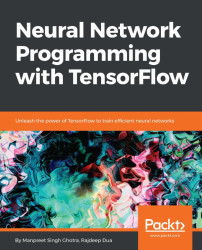•#### Neural Network Programming with Tensorflow#### Overview of this book

If you're aware of the buzz surrounding the terms such as "machine learning," "artificial intelligence," or "deep learning," you might know what neural networks are. Ever wondered how they help in solving complex computational problem efficiently, or how to train efficient neural networks? This book will teach you just that. You will start by getting a quick overview of the popular TensorFlow library and how it is used to train different neural networks. You will get a thorough understanding of the fundamentals and basic math for neural networks and why TensorFlow is a popular choice Then, you will proceed to implement a simple feed forward neural network. Next you will master optimization techniques and algorithms for neural networks using TensorFlow. Further, you will learn to implement some more complex types of neural networks such as convolutional neural networks, recurrent neural networks, and Deep Belief Networks. In the course of the book, you will be working on real-world datasets to get a hands-on understanding of neural network programming. You will also get to train generative models and will learn the applications of autoencoders. By the end of this book, you will have a fair understanding of how you can leverage the power of TensorFlow to train neural networks of varying complexities, without any hassle. While you are learning about various neural network implementations you will learn the underlying mathematics and linear algebra and how they map to the appropriate TensorFlow constructs.
Title PageCreditswww.PacktPub.comCustomer FeedbackPrefaceFree Chapter
Maths for Neural NetworksDeep Feedforward NetworksOptimization for Neural NetworksConvolutional Neural NetworksRecurrent Neural NetworksGenerative ModelsDeep Belief NetworkingAutoencodersResearch in Neural NetworksGetting started with TensorFlow## Generative models

There are two kinds of machine learning models: generative models and discriminative models. Let's examine the following list of classifiers: decision trees, neural networks, random forests, generalized boosted models, logistic regression, naive bayes, and Support Vector Machine (SVM). Most of these are classifiers and ensemble models. The odd one out here is Naive Bayes. It's the only generative model in the list. The others are examples of discriminative models.

The fundamental difference between generative and discriminative models lies in the underlying probability inference structure. In this chapter, we will study the key concepts of generative models like types and GANs, but before that, let's go through some of the key differences between generative and discriminative models.

### Discriminative versus generative models

Discriminative models learn P(Y|X), which is the conditional relationship between the target variable Y and features X. This is how least squares regression...